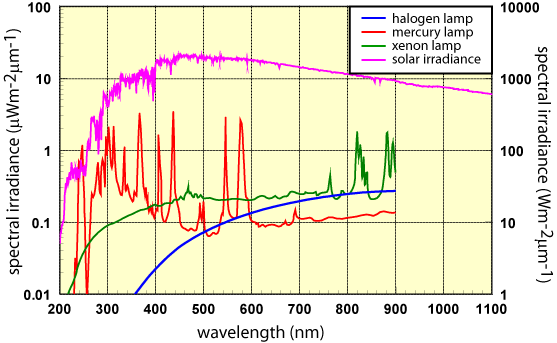Skip to main content

# 3. Spectral Irradiance

The sun is body that radiates light of many wavelengths, however there are particular wavelengths that it emits over others. This means there are photons of certain energies that the earth receives more than others. It is very helpful then to know the spectrum of light that we can capture from the sun, which we can then use to find the total energy being received from a large quantity of photons.
The spectral irradiance is given as a function of wavelength λ, and gives the power (energy per unit time) received by the surface for a particular wavelength of light. It is given by the equation:where F(λ) is the spectral irradiance in terms of photon flux Φ, energy of a given photon E, and the wavelength of the given photon λ.
This equation just means that if we multiply the photon flux (the amount of photons with a particular wavelength hitting a surface), and multiply it by the energy of the photon and divide by that wavelength, we get a measurement of how much power is coming from all the photons received per second from that particular wavelength. This gives us an idea of how much power is being contributed from each wavelength.Above we have a graph of spectral irradiance for various objects, which illustrates the power contribution from each individual wavelength of light. As you can see, lamps containing different elements give off very different spectra of light.
Source: “Spectral Irradiance”. <http://www.pveducation.org/pvcdrom/p...ral-irradiance>